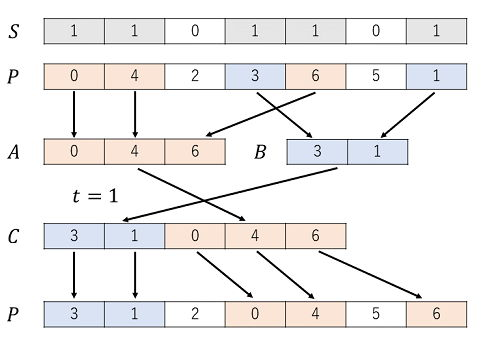시간 제한 메모리 제한 제출 정답 맞은 사람 정답 비율
2 초 512 MB 0 0 0 0.000%

## 문제

You have a permutation $P$ of length $n$. In this problem, elements of the permutation are integers from $0$ to $n - 1$.

Your task is to perform the following operation up to $30$ times to sort $P$ in ascending order.

The operation is defined by two parameters: an integer $t$ denoting the mode of operation ($0 \le t \le 1$) and a string $S$ of length $n$, consisting of 0s and 1s.

At the start of the process, we have two empty sequences, $A$ and $B$.

Next, for each $i$ from $1$ to $n$, we repeat the following step:

• If $S_i = 0$, do nothing.
• If $S_i = 1$: if $P_i$ is even, add $P_i$ to the end of sequence $A$, otherwise add $P_i$ to the end of sequence $B$.

If $t = 0$, sequence $C$ is the concatenation of sequence $A$ and sequence $B$ in that order.

If $t = 1$, sequence $C$ is the concatenation of sequence $B$ and sequence $A$ in that order.

Next, for each $i$ fron $1$ to $n$, we repeat the following step:

• If $S_i = 0$, do nothing.
• If $S_i = 1$, replace $P_i$ with the first element of $C$ and erase the first element of $C$.

For example, if $n = 7$, $P = \{0, 4, 2, 3, 6, 5, 1\}$ and we choose $t = 1$ and $S = 1101101$, the process is shown on the picture below.## 입력

The first line of the input contains one integer $n$ ($1 \le n \le 15\,000$). The second line contains $n$ integers $P_i$ ($0 \le P_i \le n - 1$, $P_i \ne P_j$ if $i \ne j$).

## 출력

Print one of the possible sorting sequences in the following format:

On the first line, print one integer $k$: the number of operations ($0 \le k \le 30$).

The $i$-th of the following $k$ lines shall describe the $i$-th operation and contain integer $t_i$ ($0 \le t_i \le 1$) and binary string $S$ of length $n$.

If there is more than one such sequence, choose any one of them. Note that you don't need to minimize $k$.

## 예제 입력 1

7
0 4 2 3 6 5 1


## 예제 출력 1

1
1 0100101